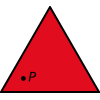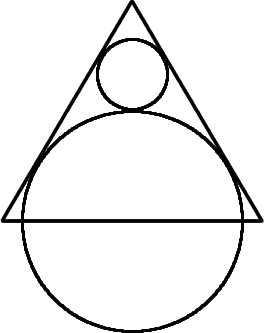#### You may also like### Fitting In

The largest square which fits into a circle is ABCD and EFGH is a square with G and H on the line CD and E and F on the circumference of the circle. Show that AB = 5EF. Similarly the largest equilateral triangle which fits into a circle is LMN and PQR is an equilateral triangle with P and Q on the line LM and R on the circumference of the circle. Show that LM = 3PQ### Triangle Midpoints

You are only given the three midpoints of the sides of a triangle. How can you construct the original triangle?### Three Way Split

Take any point P inside an equilateral triangle. Draw PA, PB and PC from P perpendicular to the sides of the triangle where A, B and C are points on the sides. Prove that PA + PB + PC is a constant.

# Tricircle

##### Age 14 to 16Challenge LevelThe centre of the larger circle is at the midpoint of one side of an equilateral triangle and the circle touches the other two sides of the triangle.

A smaller circle touches the larger circle and two sides of the triangle.

If the small circle has radius 1 unit, find the radius of the larger circle.